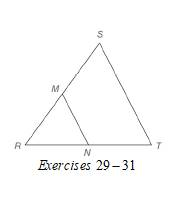Chapter 4.2, Problem 29E### Elementary Geometry for College St...

6th Edition
Daniel C. Alexander + 1 other
ISBN: 9781285195698

#### Solutions

Chapter
Section### Elementary Geometry for College St...

6th Edition
Daniel C. Alexander + 1 other
ISBN: 9781285195698
Textbook Problem
3 views

# In Exercises 29 to 31, M and N are the midpoints of sides R S ¯ and R T ¯ of ∆ R S T , respectively. Given: M N = 2 y - 3 S T = 3 y Find: y , M N , and S TTo determine

To find:

y, MN, and ST.

For MN=2y-3

ST=3y

Explanation

Calculation:

Given,

MN=2y-3

ST=3y

M N are the midpoints of sides RS¯ and RT¯ of RST respectively.

A line segment that joins the midpoints of two sides of a triangle is parallel to the third side and has a length equal to one-half the length of the third side.

Thus,

MN=12ST

2y-3=12(3y)

Multiply; by 2 on both sides,

22y-2=2·12(3y)

22y-2=3y

Apply, the distributive property of multiplication over subtraction to the left of the equation

### Still sussing out bartleby?

Check out a sample textbook solution.

See a sample solution

#### The Solution to Your Study Problems

Bartleby provides explanations to thousands of textbook problems written by our experts, many with advanced degrees!

Get Started